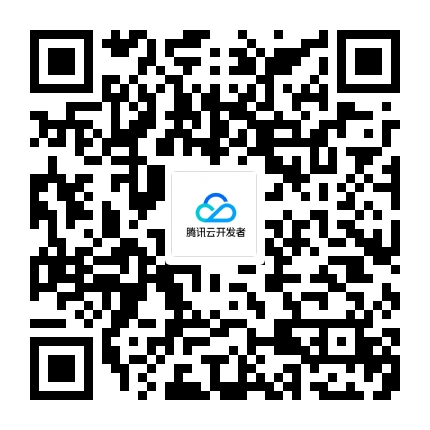TVP

# 用Swift写一个响应式编程库

2017年又快过去了，忙了一年感觉没啥收获，感觉是不是应该写点啥，想了好久没想出要写什么。下半年因为工作的原因，狗狗也没养了，吉他上也积满了灰尘，兴致勃勃的学习素描，到现在也没画出了啥??，博客也很久没更新了。想想感觉更新一下博客吧。

Reactive Programing

func fetchUser(with id: Int, completion: @escaping ((User) -> Void)) {

let user = User(name: "jewelz")

completion(user)

}

}

func fetchUser(with id: Int) -> Signal {}

fetchUser(with: "12345").subscribe({

})

enum Result {

case success(Value)

case error(Error)

}

final class Signal {

fileprivate typealias Subscriber = (Result) -> Void

fileprivate var subscribers: [Subscriber] = []

func send(_ result: Result) {

for subscriber in subscribers {

subscriber(result)

}

}

func subscribe(_ subscriber: @escaping (Result) -> Void) {

subscribers.append(subscriber)

}

}

let signal = Signal()

signal.subscribe { result in

print(result)

}

signal.send(.success(100))

signal.send(.success(200))

// Print

success(100)

success(200)

static func empty() -> ((Result) -> Void, Signal) {

let signal = Signal()

return (signal.send, signal)

}

fileprivate func send(_ result: Result) { ... }

let (sink, signal) = Signal.empty()

signal.subscribe { result in

print(result)

}

sink(.success(100))

sink(.success(200))

extension UITextField {

var signal: Signal {

let (sink, signal) = Signal.empty()

let observer = KeyValueObserver(object: self, keyPath: #keyPath(text)) { str in

sink(.success(str))

}

signal.objects.append(observer)

return signal

}

}

final class KeyValueObserver: NSObject {

private let object: NSObject

private let keyPath: String

private let callback: (T) -> Void

init(object: NSObject, keyPath: String, callback: @escaping (T) -> Void) {

self.object = object

self.keyPath = keyPath

self.callback = callback

super.init()

object.addObserver(self, forKeyPath: keyPath, options: [.new], context: nil)

}

override func observeValue(forKeyPath keyPath: String?, of object: Any?, change: [NSKeyValueChangeKey : Any]?, context: UnsafeMutableRawPointer?) {

guard let keyPath = keyPath, keyPath == self.keyPath, let value = change?[.newKey] as? T else { return }

callback(value)

}

deinit {

object.removeObserver(self, forKeyPath: keyPath)

}

}

class VC {

let textField = UITextField()

var signal: Signal?

signal = textField.signal

signal?.subscribe({ result in

print(result)

})

textField.text = "1234567"

}

deinit {

print("Removing vc")

}

}

var vc: VC? = VC()

vc = nil

// Print

success("1234567")

Removing vc

Reference Cycles

deinit {

print("Removing Signal")

}

let observer = KeyValueObserver(object: self, keyPath: #keyPath(text)) { str in

sink(.success(str))

}

static func empty() -> ((Result) -> Void, Signal) {

let signal = Signal()

return ({[weak signal] value in signal?.send(value)}, signal)

}

fileprivate typealias Token = UUID

fileprivate var subscribers: [Token: Subscriber] = [:]

final class Disposable {

private let dispose: () -> Void

static func create(_ dispose: @escaping () -> Void) -> Disposable {

return Disposable(dispose)

}

init(_ dispose: @escaping () -> Void) {

self.dispose = dispose

}

deinit {

dispose()

}

}

func subscribe(_ subscriber: @escaping (Result) -> Void) -> Disposable {

let token = UUID()

subscribers[token] = subscriber

return Disposable.create {

self.subscribers[token] = nil

}

}

map

map 比较简单，就是将一个 返回值为包装值的函数 作用于一个包装(Wrapped)值的过程， 这里的包装值可以理解为可以包含其他值的一种结构，例如 Swift 中的数组，可选类型都是包装值。它们都有重载的 map, flatMap等函数。以数组为例，我们经常这样使用：

let images = ["1", "2", "3"].map{ UIImage(named: \$0) }

func map(_ transform: @escaping (Value) -> T) -> Signal {

let (sink, signal) = Signal.empty()

let dispose = subscribe { (result) in

sink(result.map(transform))

}

signal.objects.append(dispose)

return signal

}

extension Result {

func map(_ transform: @escaping (Value) -> T) -> Result {

switch self {

case .success(let value):

return .success(transform(value))

case .error(let error):

return .error(error)

}

}

}

// Test

let (sink, intSignal) = Signal.empty()

intSignal

.map{ String(\$0)}

.subscribe { result in

print(result)

}

sink(.success(100))

// Print success("100")

flatMap

flatMap 和 map 很相似，但也有一些不同，以可选型为例，Swif t是这样定义 map 和 flatMap 的：

public func map(_ transform: (Wrapped) throws -> U) rethrows -> U?

public func flatMap(_ transform: (Wrapped) throws -> U?) rethrows -> U?

flatMap 和 map 的不同主要体现在 transform 函数的返回值不同。map 接受的函数返回值类型是 U类型，而 flatMap 接受的函数返回值类型是 U?类型。例如对于一个可选值，可以这样调用：

let aString: String? = "￥99.9"

let price = aString.flatMap{ Float(\$0)}

// Price is nil

func flatMap(_ transform: @escaping (Value) -> Signal) -> Signal {

let (sink, signal) = Signal.empty()

var _dispose: Disposable?

let dispose = subscribe { (result) in

switch result {

case .success(let value):

let new = transform(value)

_dispose = new.subscribe({ _result in

sink(_result)

})

case .error(let error):

sink(.error(error))

}

}

if _dispose != nil {

signal.objects.append(_dispose!)

}

signal.objects.append(dispose)

return signal

}

func users() -> Signal {

let (sink, signal) = Signal.empty()

sink(.success(users))

}

return signal

}

let (sink, signal) = Signal.empty()

sink(.success(User(id: id, name: "jewelz")))

}

return signal

}

let dispose = users()

.flatMap { return self.userDetail(with: \$0.first!.id) }

.subscribe { result in

print(result)

}

disposes.append(dispose)

// Print: success(ReactivePrograming.User(name: Optional("jewelz"), id: "1"))

• 发表于:
• 原文链接http://kuaibao.qq.com/s/20171209B04OII00?refer=cp_1026
• 腾讯「腾讯云开发者社区」是腾讯内容开放平台帐号（企鹅号）传播渠道之一，根据《腾讯内容开放平台服务协议》转载发布内容。
• 如有侵权，请联系 cloudcommunity@tencent.com 删除。

2023-03-25

2023-03-25

2023-03-25

2023-03-25

2023-03-25

2023-03-25

## 关注

10元无门槛代金券